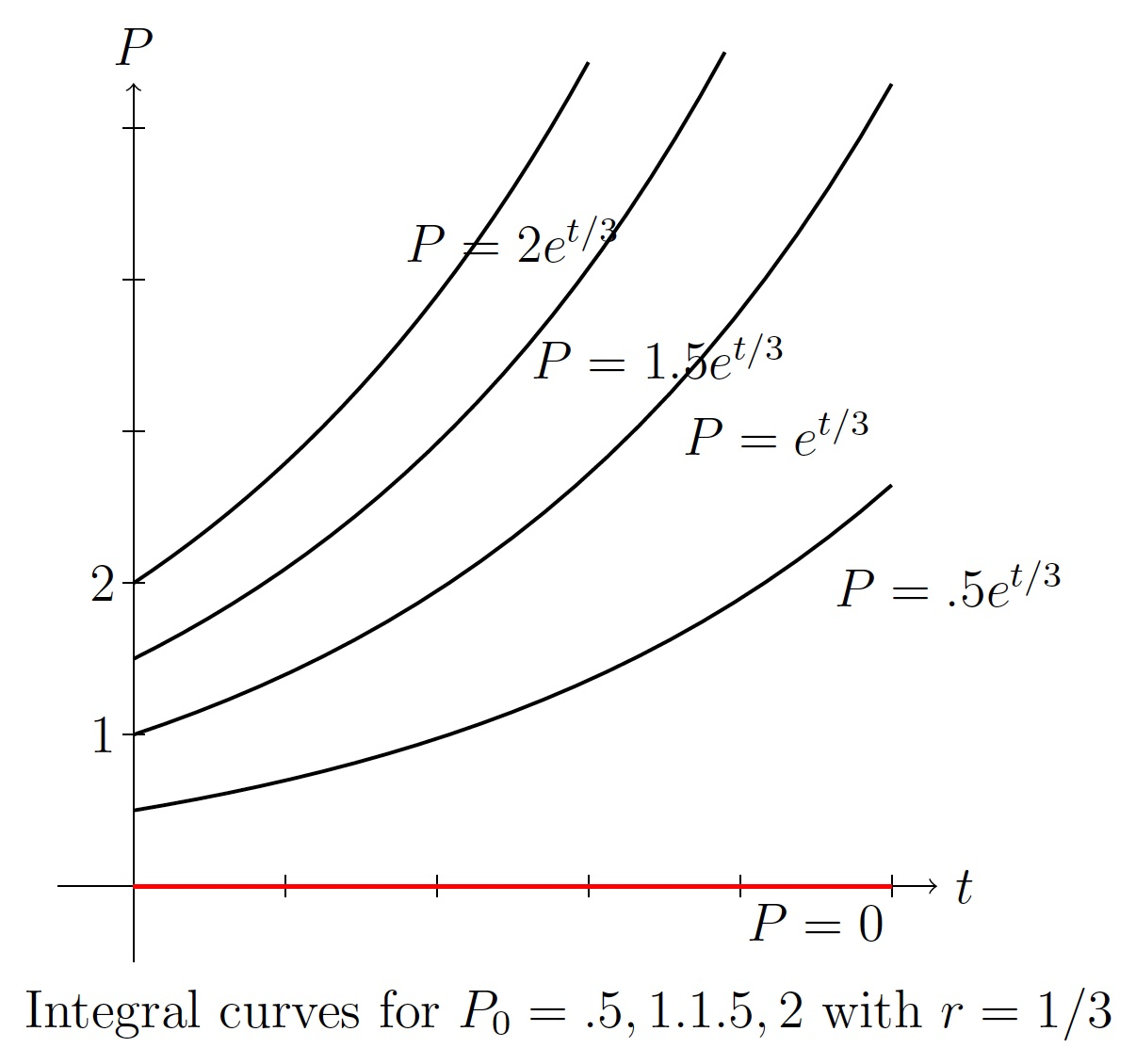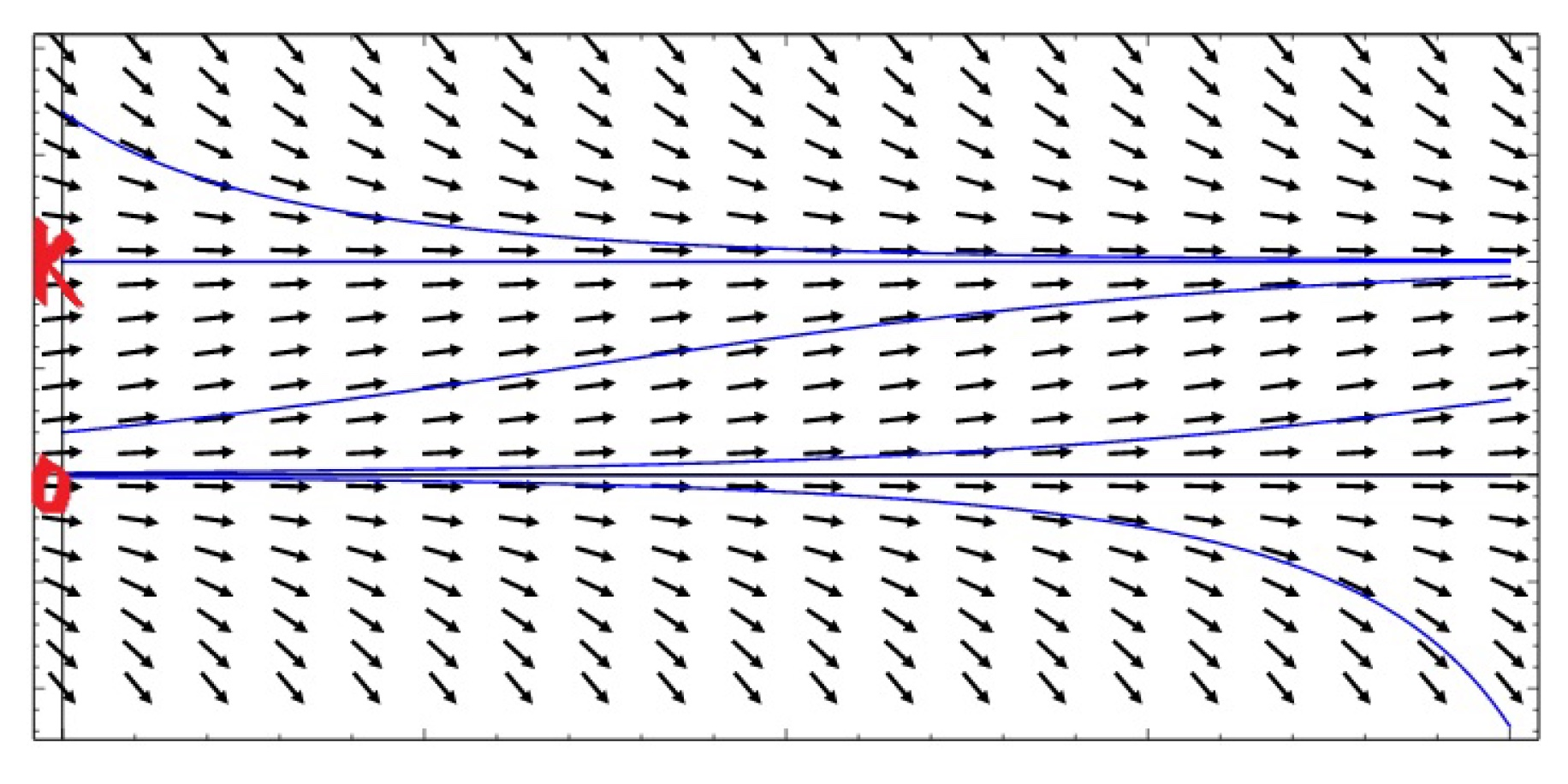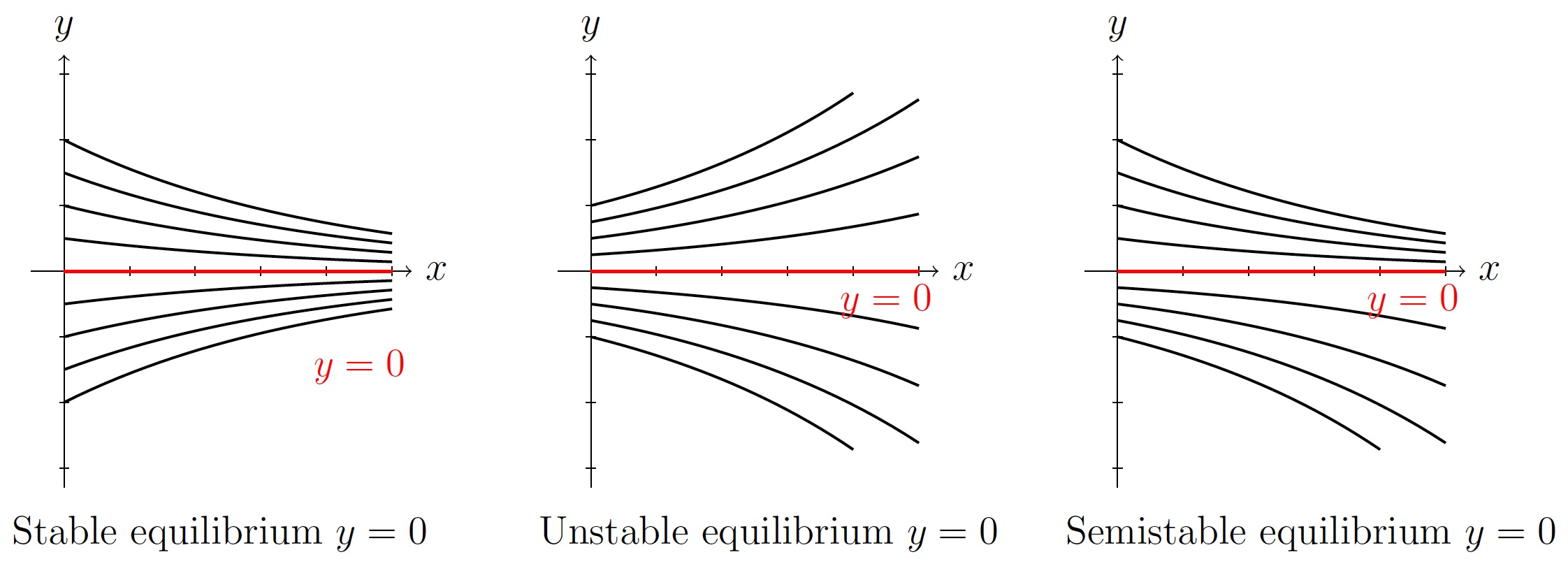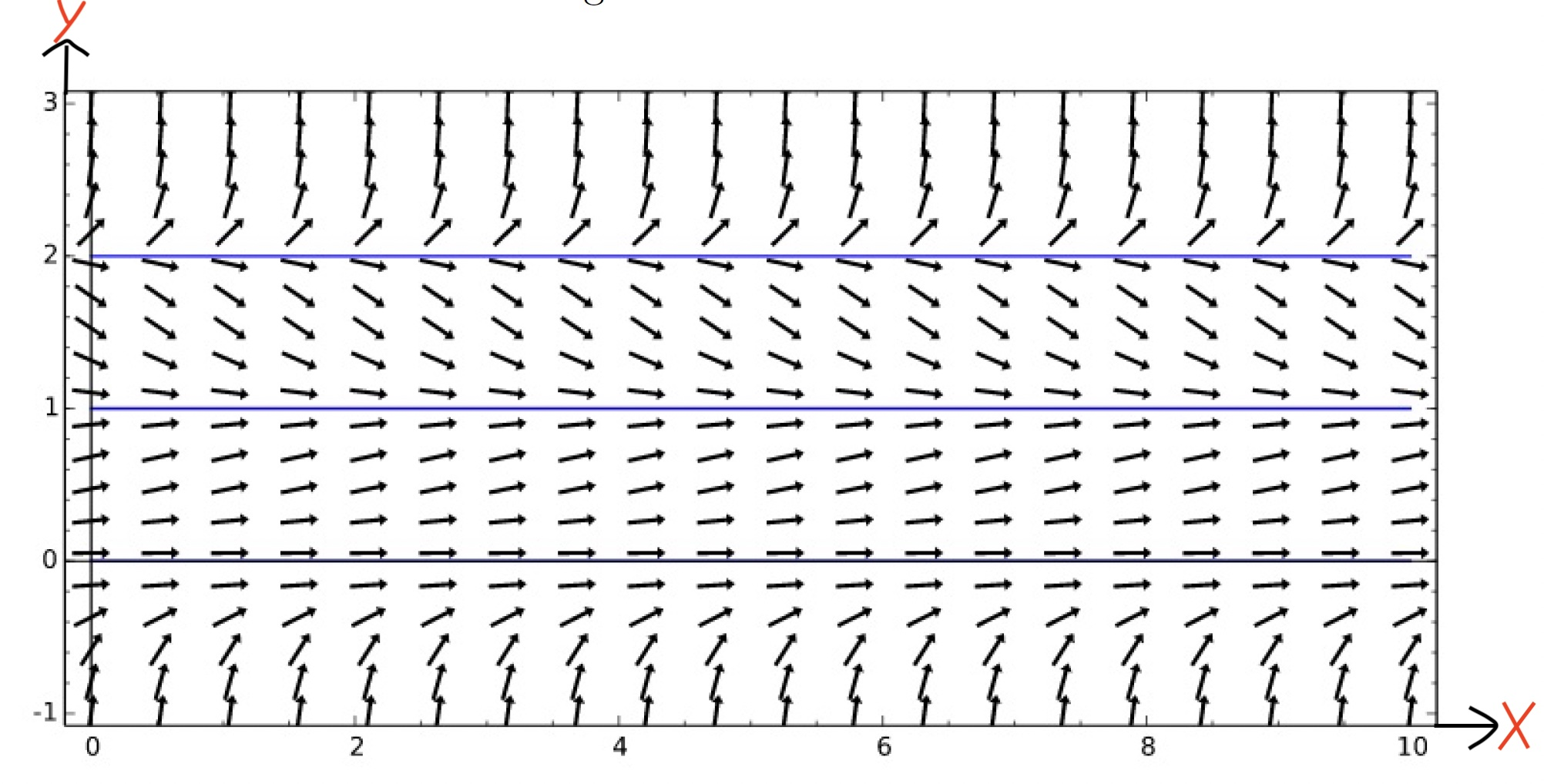DiffEq Home

## Autonomous ODEs and Stability of Equilibrium

An ODE of the form $$\displaystyle\frac{dy}{dx}=f(y)$$ is called autonomous.

Example.

1. $$\displaystyle\frac{dy}{dx}=y(y-1)$$

2. $$y'=e^y\sin y$$

3. $$\displaystyle\frac{dP}{dt}=(100-P)P$$

Recall that an equilibrium solution to an ODE is a solution $$y=g(x)$$ for which $$\displaystyle\frac{dy}{dx}=0$$. So for the autonomous ODE $$\displaystyle\frac{dy}{dx}=f(y)$$, equilibrium solutions are the solutions of $$f(y)=0$$. For example, equilibrium solutions of $$\displaystyle\frac{dy}{dx}=y(y-1)$$ are $$y=0,1$$.

Exponential population growth:
Suppose $$P(t)$$ is the population of a species at time $$t$$. The simplest assumption may be that the population growth is proportional to the current population: $\displaystyle\frac{dP}{dt}=rP \;\;\;\;(17)$ where $$r$$ is a constant representing growth when $$r > 0$$ and decline when $$r < 0$$. Solving (17) we get $$P=ce^{rt}$$ (verify). If the initial population is $$P(0)=P_0$$, then the solution to (17) is $P=P_0e^{rt}.$Note that $$P=0$$ is the only equilibrium solution to (17). Since integral curves are going away from the equilibrium solution $$P=0$$, this is called unstable equilibrium solution.

Logistic population growth:
Suppose $$P(t)$$ is the population of a species at time $$t$$. A rational assumption may be that the population will grow when the current population is small and will decline when the current population is big because of the lack of resources. There may be an ideal population size $$K$$ (called carrying capacity) such that if $$P < K$$, then population will grow and if $$P > K$$, then population will decline. In 1838 Pierre-Francois Verhulst modeled it as follows: $\displaystyle\frac{dP}{dt}=rP\left(1-\frac{P}{K}\right). \;\;\;\;(18)$ Equilibrium solutions: The ODE (18) has two equilibrium solutions $$P=0,K$$. These two equilibrium solutions have different flavors. To see this, we draw integral curves of (18) from direction field using different initial population $$P_0=P(0)$$. When $$P_0 > K$$, $$\displaystyle\frac{dP}{dt} < 0$$ and $$P\to K$$ as $$t\to \infty$$. Also when $$0 < P_0 < K$$, $$\displaystyle\frac{dP}{dt} > 0$$ and $$P\to K$$ as $$t\to \infty$$. So $$P=K$$ is a stable equilibrium as arrows (integral curves) are moving toward $$P=K$$. But arrows (integral curves) are moving away from $$P=0$$. So $$P=0$$ is a unstable equilibrium.We can verify these observations after we solve (18). \begin{align*} K\displaystyle\frac{dP}{dt}&=KrP\left(1-\frac{P}{K}\right)=rP(K-P)\\ \displaystyle\frac{K}{P(K-P)}\, dP&=r\, dt\\ \displaystyle\int\left(\frac{1}{P}+\frac{1}{K-P}\right)\, dP&=\int r\, dt\\ \ln P-\ln(K-P)&=rt+c' \end{align*} \begin{align*} \ln\left(\frac{P}{K-P} \right)&=rt+c'\\ \frac{P}{K-P}&=e^{rt+c'}=ce^{rt} \;(\text{where }c=e^{c'}) \;\;\;\;(19)\\ P&=ce^{rt}(K-P)\\ P(1+ce^{rt})&=Kce^{rt}\\ P&=\frac{Kce^{rt}}{(1+ce^{rt})}\;\;\;\;\;\;\;\;\;\;\;\;\;\;\;\;\;\;\;\;\;\;\;\;\;\;\;\;\;\;(20) \end{align*} If the initial population is $$P(0)=P_0$$, then from (19) we get $c=\frac{P_0}{K-P_0}.$ Plugging $$c$$ into (20), we get $P=\frac{KP_0e^{rt}}{K+P_0(e^{rt}-1)}$ Note that $$P=\displaystyle\frac{KP_0e^{rt}}{K+P_0(e^{rt}-1)}=\frac{K}{1+(K-P_0)e^{-rt}P_0^{-1}}\to K$$ as $$t\to \infty$$. Thus $$P=K$$ is an (asymptotically) stable equilibrium.

Steps for classifications of stability:
Suppose $$y=c$$ is an equilibrium of $$y'=f(y)$$.

1. If $$f(y) > 0$$ when $$y < c$$, and $$f(y) < 0$$ when $$y > c$$, then the equilibrium solution $$y=c$$ is stable. In this case arrows above and below $$y=c$$ are moving toward $$y=c$$.

2. If $$f(y) < 0$$ when $$y < c$$, and $$f(y) > 0$$ when $$y > c$$, then the equilibrium solution $$y=c$$ is unstable. In this case arrows above and below $$y=c$$ are moving away from $$y=c$$.

3. If $$f(y)$$ has the same sign when $$y < c$$ and $$y > c$$, then the equilibrium solution $$y=c$$ is semistable. In this case arrows above and below $$y=c$$ are moving away from $$y=c$$ from one side and moving toward $$y=c$$ from another side.Example. Find the equilibrium solutions of $$y'=y^2(y-1)(y-2)$$ and classify the stability of the equilibrium solutions.

Solution. $$y'=y^2(y-1)(y-2)=0\implies y=0,1,2$$. So equilibrium solutions are $$y=0,1,2$$. Note that $$y'=y^2(y-1)(y-2) > 0$$ when $$y > 2$$ and $$y'=y^2(y-1)(y-2) < 0$$ when $$1 < y < 2$$. Thus $$y=2$$ is an unstable solution.
Also $$y'=y^2(y-1)(y-2)<0$$ when $$1 < y < 2$$ and $$y'=y^2(y-1)(y-2) > 0$$ when $$y < 1$$. Thus $$y=1$$ is a stable solution.
But $$y'=y^2(y-1)(y-2) > 0$$ when $$y < 1$$ which implies $$y=0$$ is a semistable solution. These are also clear from the following direction field.Last edited